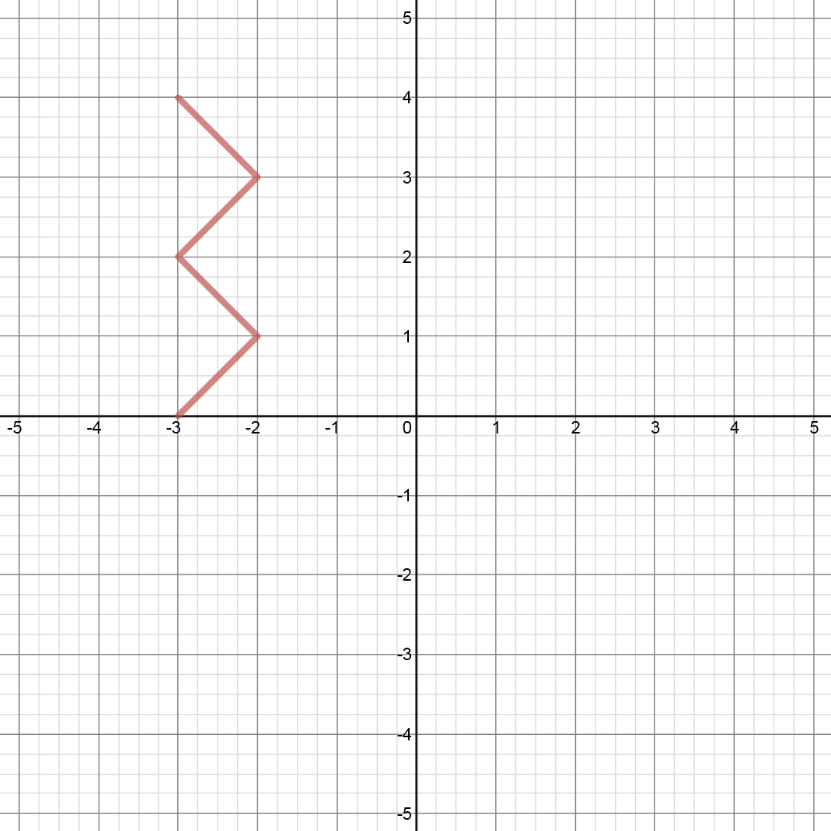# Inverse functions#### Everything You Need in One Place

Homework problems? Exam preparation? Trying to grasp a concept or just brushing up the basics? Our extensive help & practice library have got you covered.#### Learn and Practice With Ease

Our proven video lessons ease you through problems quickly, and you get tonnes of friendly practice on questions that trip students up on tests and finals.#### Instant and Unlimited Help

Our personalized learning platform enables you to instantly find the exact walkthrough to your specific type of question. Activate unlimited help now!

0/1
##### Intros
###### Lessons

1. • What is "inverse", and what does "inverse" do to a function?
• Inverse: switch "x" and "y"
• Inverse: reflect the original function in the line "y = x"
0/7
##### Examples
###### Lessons
1. Graph an inverse
Given the graph of $y = f\left( x \right)$ as shown,1. Sketch the graph of the inverse $y = {f^{ - 1}}\left( x \right)$ in 2 ways:
i) by reflecting $f\left( x \right)$ in the line $y = x$
ii) by switching the x and y coordinates for each point on $f\left( x \right)$
2. Is $f\left( x \right)$ a function?
Is ${f^{ - 1}}\left( x \right)$ a function?
2. Inverse of a Quadratic Function
Consider the quadratic function: $f(x) = (x+4)^2 + 2$
1. Graph the function $f\left( x \right)$ and state the domain and range.
2. Graph the inverse ${f^{ - 1}}\left( x \right)$ and state the domain and range.
3. Is ${f^{ - 1}}\left( x \right)$ a function?
If not, describe how to restrict the domain of $f\left( x \right)$ so that the inverse of $f\left( x \right)$ can be a function.
3. Determine the equation of the inverse.
Algebraically determine the equation of the inverse ${f^{ - 1}}\left( x \right)$, given:
1. $f\left( x \right) = - 5x + 4$
2. $f\left( x \right) = {\left( {7x - 8} \right)^3} - 1$
3. $f\left( x \right) = \frac{{3x}}{{2 + x}}$
###### Topic Notes
An inverse function is a function that reverses all the operations of another function. Therefore, an inverse function has all the points of another function, except that the x and y values are reversed.